# Three dice

The player throwing the three dice asked G. Galilei the question: "Should I bet on the sum of 11 or the sum of 12?" What did Galilei answer him?

Hint: write down all three triples of numbers that can be thrown and:
have a total of 11
have a total of 12

and compare probabilities.

Correct result:

p11 =  0.125
p12 =  0.1157
v =  11

#### Solution: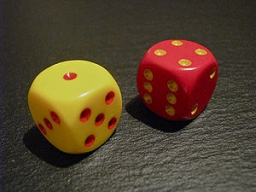We would be pleased if you find an error in the word problem, spelling mistakes, or inaccuracies and send it to us. Thank you!Tips to related online calculators
Would you like to compute count of combinations?

## Next similar math problems:

• What is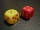What is the probability that the sum of 9 will fall on a roll of two dice? Hint: write down all the pairs that can occur as follows: 11 12 13 14 15. . 21 22 23 24. .. . 31 32. .. . . . . . .. . 66, count them, it's the variable n variable m: 36, 63,. .. .
• Sum or productWhat is the probability that two dice fall will have the sum 7 or product 12?
• Sum on diceWe have two dice. What is the greater likelihood of fall a total sum 7 or 8 ? (write 7, 8 or 0 if the probabilities are the same)?
• Three-digit numbersWe have digits 0,1,4,7 that cannot be repeated. How many three-digit numbers can we write from them? You can help by listing all the numbers.
• Six on diceWhat is the probability that when throwing two dice will fall at least one six?
• Fall sum or sameFind the probability that if you roll two dice, it will fall the sum of 10, or the same number will fall on both dice.
• DicesWe will throw two dice. What is the probability that the ratio between numbers on first and second dice will be 1:2?
• Three dice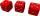What is the probability that when we roll three dice, we roll the numbers 1,2,3?
• One threeWe throw two dice. What is the probability that max one three falls?
• Sum 10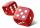What is the probability that two dice throw twice in a row will result the sum of 10?
• Three numbersWe have three different non-zero digits. We will create all 3 digits numbers from them to use all 3 figures in each number. We add all the created numbers, and we get the sum of 1554. What were the numbers?
• ThrowWe throw 2 times with 2 dices. What is the probability that the first roll will fall more than sum of 9 and the second throw have sum 3 or does not have the sum 4?
• Dice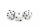How many times must throw the dice, the probability of throwing at least one six was greater than 90%?
• The diceWhat is the probability of events that if we throw a dice is rolled less than 6?
• The testThe test contains four questions, and there are five different answers to each of them, of which only one is correct, the others are incorrect. What is the probability that a student who does not know the answer to any question will guess the right answer
• Boys and girlsThere are 11 boys and 18 girls in the classroom. Three pupils will answer. What is the probability that two boys will be among them?
• Games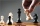Jack and Paul decided to play chess against each other. They bet ten pesos on each game they played. Jack won three bets and Paul won fifty pesos. How many games did they play?